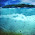## Java program to find sum of 2 number using method

simple example program for java method

java program to find sum using method
Program:-

Method 1
Java program to find sum of 2 number using method

import java.util.Scanner;

class group{
public static void main(String arng[]){
Scanner data = new Scanner(System.in);
int num1, num2;
System.out.println("Enter 1st number");
num1 = data.nextInt();
System.out.println("Enter 2nd number");
num2 = data.nextInt();

sum(num1,num2);

}
// sum method
public static void sum(int numx, int numy){
int sum=0;
sum=numx+numy;
System.out.println("Sum of 2 number:"+sum);

}
}

Output:-

Enter 1st number
2
Enter 2nd number
3
Sum of 2 number:5

Method 2
Java program to find sum of 2 number using method with return value

import java.util.Scanner;

class group{

public static void main(String anrg[]){
Scanner data = new Scanner(System.in);
int num1, num2, sum;

System.out.println("Enter 1st number");
num1=data.nextInt();

System.out.println("Enter 2nd number");
num2=data.nextInt();

sum=total(num1, num2);
System.out.println("The total "+sum);
}
// total method
public static int total(int x, int y)
{
int s;
s = x+y;
return s;
}
}

Output:-

Enter 1st number
20
Enter 2nd number
15
The total 35

Learn Java:-
Java codes

Java Programs:-
List of Java Programs

1.2.any another method...?

3.thank u i am very confused abt return value concept now i understand...:)

4.public class Function1 {
public static void main(String[] args) {
// TODO Auto-generated method stub
int a=10;
int b=2;
int d= sub(a,b);
System.out.println(c);
System.out.println(d);

}
private static int Add(int x, int y) {

}
public static int sub( int r, int s){
int sub= r-s;
return sub;
}
}

5.public class Function1 {
public static void main(String[] args) {
// TODO Auto-generated method stub
int a=10;
int b=2;
int d= sub(a,b);
System.out.println(c);
System.out.println(d);

}
private static int Add(int x, int y) {

}
public static int sub( int r, int s){
int sub= r-s;
return sub;
}
}

6.Nice post covered both the aspects with return and without return type. One suggestion please indent the codes properly. It would make it look clean.
My blog post : Short article on how to learn java programming

7.its very helpful thanks

8.In first program output is not showing it just accept/read two integer values but not showing addition of them